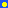Sidebar

# Density

Density is a way to measure how tightly packed an object is. Denser objects have less empty space in them, they are less holey. The density of an object is often measured in grams per milliliter. So to measure the density of an object you would put it on a balance to measure how many grams it is (the object's mass) then divide that number by the amount of space the object takes up (its volume). The density of something stays the same wherever you take it, on Earth, Mars, or anywhere in the universe.

So how could you change the density of something? Let's say you are outside in the snow and want to make a snowball. You take some snow and pact it together until you have a snowball about the size of a grapefruit. Then without adding any more snow, you squash your snowball some more until it is the size of an orange. The orange sized snowball is more dense than the grapefruit sized snowball because it has the same amount of snow in it, but takes up less space. So to increase the density of something you could decrease the amount of space it takes up, as long as you keep everything else the same (the amount of stuff and the kind of stuff you have).

The density of an object compared to the density of water tells us whether the object will float or not. The density of water is about 1.00 g/mL. If the density of an object is less than the 1.00 g/mL, the object will float in water. If the density of something is greater than 1.00 g/mL it will sink in water.

Have you ever had ice water before? If you have, you'll probably remember that the ice cubes float in water. That's because ice is less dense than water. When you freeze water to make ice, the water expands and takes up more space. So a piece of ice has more volume than the same amount of water. Since ice has more volume for the same amount of stuff it makes sense that it is less dense than water and floats!Here are some densities of different things. The units are grams per cubic centimeter. A cubic centimeter is about a thimblefull and a gram is a little bit more than the weight of an M & M.

Water = 1.0Ice = 0.9People about 1.0Lead = 11.3Mercury = 13.6Air = 0.0013

Remember: things that float have densities less than 1. Things that sink have densities greater than 1.